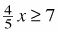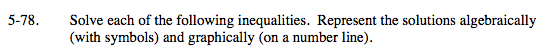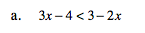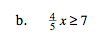### Home > MC2 > Chapter 5 > Lesson 5.2.4 > Problem5-78

5-78.

Solve each of the following inequalities. Represent the solutions algebraically (with symbols) and graphically (on a number line). Homework Help ✎

1. 3x – 4 < 3 – 2x

2.Solve each inequality like you would solve any equation.
Solve for the variable by isolating the variables and number values.

3x − 4 < 3 − 2x
+ 2x + 2 x

$x \ge \frac{35}{4}$

Note: Steps 1 and 2 can happen in any order.

5x − 4 < 3
+4 +4
5x + 0 < 7

Simplify.

5x < 7

$\frac{5x}{5} \text{ < } \frac{7}{5}$

Use this simplified inequality to create a number line similar to problem 4-68.
Now try part (b) using the same strategy.